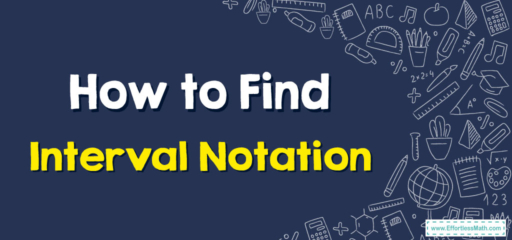# How to Find Interval Notation

Interval notation provides a succinct way to describe sets of numbers, letting you quickly understand which numbers are included and excluded from a set. In this article, we will learn together how to find interval notation.## Step-by-step Guide to Find Interval Notation

Here is a step-by-step guide to finding interval notation:

### Step 1: A Grasping of Boundaries: Understand the Basics.

• Endpoints: In the realm of numbers, endpoints are the alpha and omega of our interval. They’re the sentinels, defining where your interval starts and concludes.
• Types of Endpoints: Behold the distinction – the “closed” endpoint includes the value (marked as $$[a]$$ or $$[b]$$) while the “open” endpoint excludes it (represented as $$(a$$ or $$b)$$).

### Step 2: Dive into the Depths: Identify the Interval.

• Visual Analysis: Stare at the given number line, graph, or mathematical problem. Can you discern where the interval begins and culminates?
• Determine Continuity: Does the interval span a continuous range? Or do you encounter islands of isolated numbers?

### Step 3: Enumerate the Realm: Identify the Type of Interval.

• Infinite Expanses: Does the interval stretch indefinitely? Note these infinite possibilities:
• $$∞$$: The symbol that strides endlessly towards the positive spectrum.
• $$−∞$$: This one drifts eternally in the negative abyss.
• Finite Confinements: Are you dealing with a limited range? Ah, that’s when both endpoints are tangible numbers.

### Step 4: The Dance of Inclusivity: Determine Open or Closed Intervals.

• Gaze at the Graph: On a number line:
• A filled dot indicates the embrace of that value – a closed interval.
• An empty dot showcases exclusion, hinting at an open interval.
• In Mathematical Statements: Phrases such as “less than or equal to” beckon a closed interval. In contrast, “less than” (without equality) points towards an open interval.

### Step 5: The Grand Conjunction: Unions.

• Disjointed Intervals: Sometimes, intervals can be discrete, disconnected fragments.
• The Bridging Symbol: The union symbol $$∪$$ serves to connect these fragments in your notation.

### Step 6: The Art of Transcription: Writing the Interval Notation.

• The Basic Syntax: The common structure is a​, b​, where the underlines can be either brackets $$[ ]$$ or $$($$.
• Incorporating Infinity: For infinite intervals, replace one or both endpoints with $$∞$$ or $$−∞$$. Always use parentheses with infinity; it’s a concept, not a precise number.

• Consistency: Ensure that the notation accurately reflects the interval’s nature.
• Validity: Confirm that you’ve appropriately used brackets or parentheses to indicate open or closed intervals.

### Step 8: Embark on Further Journeys: Apply and Practice.

• Expand Horizons: Delve into more complex scenarios, such as absolute value inequalities.
• Practice is the Key: The universe of numbers is vast. Wander frequently to familiarize yourself with its intricacies.

By mastering this opulent and detailed procedure, you’ll be well-equipped to navigate the rich tapestry of interval notation. The more you delve into its complexities, the more rewarding your mathematical adventures will become!

### What people say about "How to Find Interval Notation - Effortless Math: We Help Students Learn to LOVE Mathematics"?

No one replied yet.

X
30% OFF

Limited time only!

Save Over 30%

SAVE $5 It was$16.99 now it is \$11.99